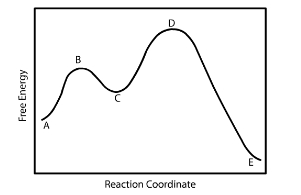Problem: A reaction coordinate diagram is shown below for the reaction of A to form E. Answer the following questions.i) Identify the transition state(s)?ii) Which is the fastest reaction? iii) Identify the rate limiting step?iv) Which compound is the most unstable?

FREE Expert Solution
92% (303 ratings)
Problem Details

A reaction coordinate diagram is shown below for the reaction of A to form E. Answer the following questions.

i) Identify the transition state(s)?

ii) Which is the fastest reaction?

iii) Identify the rate limiting step?

iv) Which compound is the most unstable?What scientific concept do you need to know in order to solve this problem?

Our tutors have indicated that to solve this problem you will need to apply the Energy Diagrams concept. You can view video lessons to learn Energy Diagrams Or if you need more Energy Diagrams practice, you can also practice Energy Diagrams practice problems .

What is the difficulty of this problem?

Our tutors rated the difficulty of A reaction coordinate diagram is shown below for the reactio... as medium difficulty.

How long does this problem take to solve?

Our expert Chemistry tutor, Dasha took 4 minutes to solve this problem. You can follow their steps in the video explanation above.

What professor is this problem relevant for?

Based on our data, we think this problem is relevant for Professor Lanni's class at SCAR.